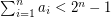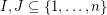Importance: High ✭✭✭
 Author(s):
 Subject: Theoretical Computer Science » Complexity
 Keywords: polynomial algorithm search problem
Problem   Letbe natural numbers with. It follows from the pigeon-hole principle that there exist distinct subsetswith. Is it possible to find such a pairin polynomial time?
This is one of a class of search problems for which a positive solution is garaunteed (so the corresponding decision problem is trivial) based on a theoretical property of the problem. Another such problem is given a Hamiltonian cycle in a cubic graph, find a second Hamiltonian cycle (here a theorem of Smith guarantee's a positive solution). The above problem is particularly attractive, since the proof that a pairmust exist is quite simple, but it gives no insight into how to find the pair.